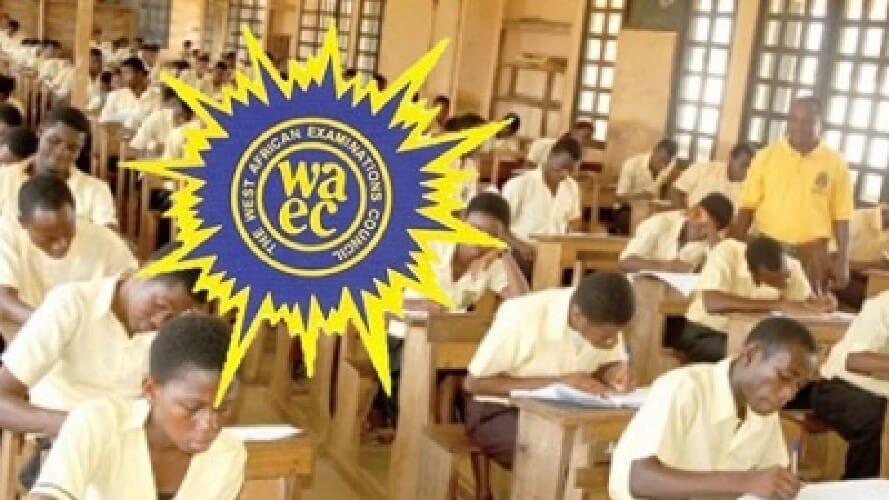# WASSCE 2023 Integrated Science Questions for WASSCE CandidatesWASSCE 2023 Integrated Questions for WASSCE Candidates. These are trial questions to help candidates check if they are ready for such questions in the upcoming WASSCE for school candidates.

## WASSCE 2023 Integrated Science Questions for WASSCE Candidates

1.(a) (i) What is an inorganic fertilizer?
(ii) Give three examples of inorganic fertilizers that could be used in maize production.

(b) State:

(i) two industrial uses each of the following metals:
(α) aluminum;
(β) copper
(b) (ii) Three reasons why gold is regarded as a metal.

c) A force acts on a body of mass 15 g and causes the body to move with acceleration of 40.0 ms-2. Calculate the force acting on the body.

(d) (i) Give one reason why trypanosome is considered as a parasite.
(d) (ii) State two ways of controlling trypanosomiasis

(2) (a) Write the IUPAC names of each of the following compounds:
(i) FeS;
(ii) CH3CH2COOH;
(iii) H2SO3;
(iv) CH3CHClCH3

(b) (i) List three organelles found in an animal cell
(ii) State one function of each of the organelles listed in (b) (i).

(c) State three reasons each for carrying out each of the following husbandry practices in poultry production:
(i) identification;
(ii) debeaking
(ii) Name two radioisotopes used in medicine.

3.(a) A student weighed 7.5 g of NaHCO3 in the laboratory.
(i) Name the apparatus used.
(ii) Calculate the number of moles of the substance weighed
[Na = 23, 0 = 16, C = 12, H =1]
(b) Explain how each of the following respiratory structures in humans is adapted to its function:
(i) nasal chamber;
(ii) diaphragm;
(iii) alveoli.
(c) Suggest five factors that could contribute to cannibalism in a poultry house.
(d) (i) Define mechanical advantage as applied to simple machines.
(ii) A load of 200N is lifted by a force of 400 N. Calculate the mechanical advantages

(4) (a) Describe the processes involved when the eye of a human views an object.

(b) (i) (α) What is an unsaturated hydrocarbon?
(β) Give two examples of unsaturated
(ii) Write down the structural formula for 2-methylpentane.

(c) State two benefits each of the following practices in animal production:
(i) dehorning;
(ii) drenching

(d) (i) Explain why a girl in a classroom could see clearly the writing on the board from the back seat but not from the front seat.
(ii) Suggest the appropriate remedy to the problem stated in (d) (i).

## WASSCE 2023 Integrated Questions for WASSCE Candidates

5.(a) State:
(i) Four physical properties of water.
(ii) The reason why water is referred to as a universal solvent.

(b) (i) Name two gases which contribute to greenhouse effect.
(b)(ii) State three effects of greenhouse on climate.

(c) (i) What is weathering as used in soil formation?

(c)(ii) Explain how hydration aids in the weathering of rocks?

(d)(i) State the energy transformation involved in hydroelectric power generation

(d)(ii) Explain why the hydroelectric power dam has thicker walls at the base than at the top.

(6)(a) (i) What is heat?

(ii) State three effects of heat on a substance.

(b)(i) Classify each of the following substances as acidic, or basic or neutral:
(i) sodium chloride;
(ii) tomato juice;
(iii) vinegar;
(iv) sea water;
(v) bicarbonate of sodium.

(c) A man with blood group B is married to a woman with blood group B. The woman gave birth to a child with blood group AB. With the aid of genetic diagram, determine whether the man is the father of the child.

(d) State four factors a farmer should consider when selecting rabbits for breeding.